# Chemistry Test 6 - Redox Reaction, Nitrogen, Oxygen Family, Carboxylic Acid, Amines Containing Nitrogen Compounds

## 25 Questions MCQ Test Mock Test Series for JEE Main & Advanced 2022 | Chemistry Test 6 - Redox Reaction, Nitrogen, Oxygen Family, Carboxylic Acid, Amines Containing Nitrogen Compounds

Description
Attempt Chemistry Test 6 - Redox Reaction, Nitrogen, Oxygen Family, Carboxylic Acid, Amines Containing Nitrogen Compounds | 25 questions in 60 minutes | Mock test for JEE preparation | Free important questions MCQ to study Mock Test Series for JEE Main & Advanced 2022 for JEE Exam | Download free PDF with solutions
QUESTION: 1

### System which suggested Roman numbers to name compounds is called as

Solution: Stock nomenclature for inorganic compounds is a widely used system of chemical nomenclature developed by the German chemist Alfred Stock and first published in 1919.
QUESTION: 2

Solution:
QUESTION: 3

### Suppose a compound contains atoms A,B and C. Theoxidation number of A is 2, B is 5 and C is –2.The possible formula of the compound would be

Solution: When we calculate oxidation number sum should come as zero In c option 2*3+5*2+8*-2=0
QUESTION: 4
Among the following, the strongest oxidising agent is
Solution:
QUESTION: 5
For the redox reaction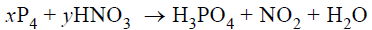Solution:
QUESTION: 6
The oxidation number of sulphur in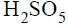is
Solution:
QUESTION: 7
The equivalent weight of Nitrogen in the reaction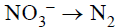is
Solution:
QUESTION: 8
Which of the following ions will undergo
disproportionation reaction ?
Solution:
QUESTION: 9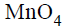is good oxidizing agent in different media,where it changes to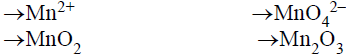The oxidatino number of Mn correspondingly reduces by
Solution:
QUESTION: 10
In the complex Ion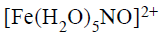Solution:
QUESTION: 11
The IUPAC name of the complex compound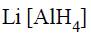is
Solution: The ionization sphere which is lithium Then inside the complex entity u will start with the ligand, therefore, tetrahydride(Because there are 4 hydrogens) and then comes the metal aluminate oxidation number of aluminum is 3. Therefore the name of the compound will be lithium tetrahydride aluminate(III)
QUESTION: 12
The IUPAC name for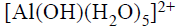is
Solution:
QUESTION: 13
The hyridization states of the central atom in the complex
ions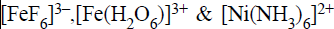are
Solution:
QUESTION: 14
Which of the following statements is correct ?
Solution:
QUESTION: 15
Consider the following spatial arrangements of the
octahedral complex ion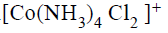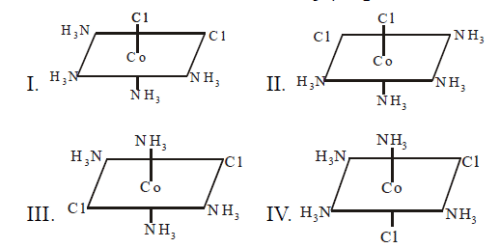Which of the following statement is incorrect
regarding these stuructures ?
Solution:
QUESTION: 16
The chloride ion is detected by the formation of a white
precipitate of AgCl on addition of aqueous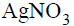solution and by the dissociation of the precipitate on
addition of excess ammonia solution. AgCl dissolves in
aqueous ammonia due to the formation of water woluble
Solution:
QUESTION: 17
One mole of the complex compound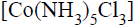gives 3 moles of ions on dissolution in water. One mole of the same complex reacts with two moles ofsolution to yield two moles of AgCl(s).The structure of the complex is
Solution:
QUESTION: 18
Which of the following have the general formula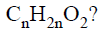Solution:
QUESTION: 19
Which of the following compounds on heating at about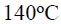gives acetic acid ?
Solution:
QUESTION: 20
In which of the following reactions does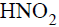act as oxidizing agent ?
Solution:
*Answer can only contain numeric values
QUESTION: 21

A compound of Xe and F is found to have 53.5% Xe. What is the oxidation number of Xe in this compound? (At. wt. of Xe = 131):-

Solution: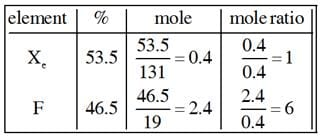Hence compound may be XeF6.

*Answer can only contain numeric values
QUESTION: 22

How many species out of the following are aromatic?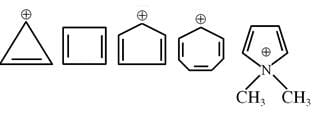Solution: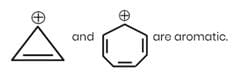*Answer can only contain numeric values
QUESTION: 23

Amongst the following, the total number of nucleophiles is -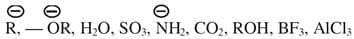Solution:

L.p or – Ve charge having compounds are nucleophiles.

*Answer can only contain numeric values
QUESTION: 24

How many πe in given structure?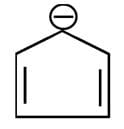Solution:
*Answer can only contain numeric values
QUESTION: 25

How many of the following give positive isocyanide test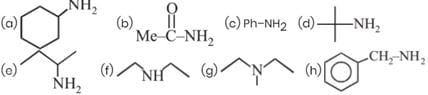Solution:

a, c, d, e, hUse Code STAYHOME200 and get INR 200 additional OFF Use Coupon Code